Computers, Materials & ContinuaDOI:10.32604/cmc.2022.021072Article

MLA: A New Mutated Leader Algorithm for Solving Optimization Problems

1Department of Mathematics and Computer Sciences, Sirjan University of Technology, Sirjan, Iran
2Graduate of Department of Electrical and Electronics Engineering, Shiraz University of Technology, Shiraz, Iran
3Department of Electrical and Electronics Engineering, Shiraz University of Technology, Shiraz, Iran
4Department of Mathematics, Faculty of Science, University of Hradec Králové, 500 03, Hradec Králové, Czech Republic
5Department of Computer Science, Government Bikram College of Commerce, Patiala, Punjab, India
*Corresponding Author: Pavel Trojovský. Email: pavel.trojovsky@uhk.cz
Received: 22 June 2021; Accepted: 23 July 2021

Abstract: Optimization plays an effective role in various disciplines of science and engineering. Optimization problems should either be optimized using the appropriate method (i.e., minimization or maximization). Optimization algorithms are one of the efficient and effective methods in providing quasi-optimal solutions for these type of problems. In this study, a new algorithm called the Mutated Leader Algorithm (MLA) is presented. The main idea in the proposed MLA is to update the members of the algorithm population in the search space based on the guidance of a mutated leader. In addition to information about the best member of the population, the mutated leader also contains information about the worst member of the population, as well as other normal members of the population. The proposed MLA is mathematically modeled for implementation on optimization problems. A standard set consisting of twenty-three objective functions of different types of unimodal, fixed-dimensional multimodal, and high-dimensional multimodal is used to evaluate the ability of the proposed algorithm in optimization. Also, the results obtained from the MLA are compared with eight well-known algorithms. The results of optimization of objective functions show that the proposed MLA has a high ability to solve various optimization problems. Also, the analysis and comparison of the performance of the proposed MLA against the eight compared algorithms indicates the superiority of the proposed algorithm and ability to provide more suitable quasi-optimal solutions.

Keywords: Optimization; metaheuristics; leader; benchmark; objective function

1  Introduction

Nowadays, by enhancing information technology, various number of optimization problems arises in several fields such as bioinformatics, operation research, geophysics, and engineering etc [1,2]. Hence, optimization has become a fundamental issue that plays a remarkable role in a wide range of programs and daily life. The simple concept of optimization is a method for obtaining the favorable solution for a problem by optimizing (i.e., minimizing or maximizing) a function in terms of some variables. In general, optimization problems consist of three main parts, including objective function, constraints, and optimization variables . Generally, methods for solving optimization problems can be divided into two categories, included deterministic and stochastic methods .

Deterministic methods, which are gradient-based methods and depending on the nature of the equations and variables, i.e., Simplex, Branch, Bound, Non-linear methods, etc. Deterministic algorithms are able to precisely find the optimal solutions, but they are not suitable for complicated and intractable problems, and their responding time grows exponentially in such complicated problems . Unfortunately, most real-life optimization problems include large solution space, non-convex, and non-linear objective functions and constraints. Such optimization problems classified as NP-hard problems and have high computational complexity that couldn't be solved in a polynomial time and complicated in nature. Should be noted that mathematical methods are only effective in solving small-scale problems, and not efficient and cost-effective for solving complex problems [5,6]. To tackle this problem, various approximation methods were proposed by the researchers that able to obtain an acceptable solution in a sensible time.

Compared to the gradient-based methods, they are less likely to be trapped in local optima, the gradient of the objective function is not required and can be used in high-complex and high-dimensional problems. Other main advantages of these algorithms can be mentioned as:

Being severely robust, having a high chance to find global or a near-optimum within a reasonable time, being easy to implement, and being well suitable for the discrete type of optimization problems. The big disadvantage related to these algorithms is high computational complexity, week constraint-handling capability, problem-specific parameter tuning, and limited problem size .

These stochastic methods can be categorized into two general groups: heuristics and metaheuristics. The base of stochastic methods is repetition, in which they first generate an initial population and in each iteration of the algorithm, they improve their solution until the convergence criterion satisfied .

Two major drawbacks of the heuristic algorithms are (1) Stuck with a high probability of local optimum and (2) Severe weakness in practical applications for complex and high dimensional problems . Metaheuristic algorithms are presented to overcome these shortcomings associated with heuristic algorithms. The point about stochastic methods and optimization algorithms is that optimization algorithms do not guarantee the proposed solution as a global optimal solution. However, the solutions obtained from the optimization algorithms are close to the global optimal and are therefore called quasi-optimal. For this reason, various optimization algorithms are introduced by scientists to provide better quasi-optimal solutions to optimization problems. In this regard, optimization algorithms are applied in various fields in the literature such as energy , protection , electrical engineering , and energy carriers [21,22] to achieve the optimal solution.

The contribution and innovative of this study are in introducing a new optimization algorithm called Mutated Leader Algorithm (MLA) in order to solve various optimization problems. Using the mutated leader to update the position of the algorithm population in the search space is the main idea of the proposed MLA. In addition to information about the best member of the population, the mutated leader also contains information about the worst member of the population, as well as ordinary members of the population. The proposed MLA is mathematically modeled and evaluated on a standard set consisting of twenty-three standard objective functions. In addition, the performance of the MLA in solving these objective functions is compared with eight well-known algorithms.

The rest of the article is organized in such a way that in Section 2, a study on optimization algorithms is presented. The proposed MLA is introduced in Section 3. Simulation studies are presented in Section 4. Finally, conclusions and several suggestions for further studies are provided in Section 5.

2  Background

The development of metaheuristic algorithms (such as Particle Swarm Optimization (PSO) , Genetic Algorithm (GA) , and Gravitation Search Algorithm (GSA) ) has attracted more attention from researchers and more emphasis has given to the development of these algorithms. The advantages of metaheuristics algorithms can be mentioned as simplicity and flexibility, avoidance of local optimization, high performance, derivation-free mechanism, and simplicity by nature. Metaheuristic algorithms are basically inspired by real-world phenomena and simple principles in nature to find desired solutions for optimization problems by simulating biological phenomena or physical rules. There are two main categories for Metaheuristic algorithms: evolutionary-based methods and swarm-based techniques. Generally, swarm-based techniques simulate physical phenomena and use mathematical methodologies. On the other hand, 2 originate from the natural process of biological evolution. evolutionary-based methods originate from the process of biological evolution in nature. These methods have attracted more attention from many researchers in the last decades their capability to rapidly explore the feasible space and independence from the nature of the problem.

Based on inspiration source, which is known as one of the most popular classification criteria, optimization algorithms can be categorized into four general categories as (i) swarm-based (SB), (ii) evolutionary-based (EB), (iii) physics-based (PB), and (iv) game-based (GB) methods.

2.1 Physics-Based Algorithms

Physics-based algorithms are a type of algorithm that is inspired by existing physical laws and simulates them. For instance, Simulated Annealing (SA) is inspired by the process of gradual heating and refining of metals . Specifically, SA is the metaheuristic algorithm to approximate global solutions in a large search space defined for an optimization problem. This method frequently employed for problems with discrete search space (namely the traveling salesman problem). For problems where finding an approximate global optimum is more significant than obtaining an exact local solution within a specified time, SA may be preferred in comparison to accurate algorithms such as branch and bound or gradient descent and so on. Spring Search Algorithm (SSA) is inspired by Hooke's law . This algorithm can be utilized to solve single-objective constrained optimization problems. The weights that are connected by springs are considered as the search agents of this algorithm in the search space of problem, which according to Hooke's law, the force of these springs (or equivalently search agents), is proportional to the length of each spring. GSA simulated the law of gravity, mass interactions and motion. A set of masses that interact with each other based on Newtonian gravity force and the laws of motion are considered as searcher agents in this algorithm. This force leads to a global movement of all agents towards objects with weighty masses. Accordingly, masses collaborate using a directly gravitational force. Small World Optimization Algorithm (SWOA) , Designed by small-world phenomenon process, Curved Space Optimization (CSO)  based on principles of general relativity theory, Ray Optimization (RO)  algorithm, Black Hole (BH) , Momentum Search Algorithm (MSA) , Artificial Chemical Reaction Optimization Algorithm (ACROA) , Charged System Search (CSS) , Galaxy-based Search Algorithm (GbSA) , and Magnetic Optimization Algorithm (MOA)  are other examples of this type of algorithms.

2.2 Evolution-Based Algorithms

Evolution-based algorithms are one more type of optimization algorithms that utilize mechanisms inspired by biological evolution, such as mutation, reproduction, selection, and recombination. Algorithms such as GA, Biogeography-based Optimizer (BBO) , Differential Evolution (DE) , Evolution Strategy (ES) , and Genetic Programming (GP)  are belong to this group. For instance, GA is one of the well-known evolutionary-based metaheuristic algorithms. This algorithm is inspired by the natural selection process. GA commonly utilized to generate high-quality answers to optimization problems relying on biologically inspired operators namely, mutation, selection, crossover, and so on. BBO is generally employed to optimize multi-dimensional functions, but it does not apply the gradient of the function, which means that it does not need the function to be differentiable as required by classic optimization algorithms (namely newton and gradient descent algorithms). Hence, BBO can be used on discontinuous functions. Evolutionary Strategy (EA) is inspired by natural selection deals with a division of population-based optimization algorithms. According to natural selection theory individuals with characteristics useful to their survival are able to live through generations and transfer the good features to the next generation.

2.3 Swarm -Based Algorithms

Swarm-based algorithms are another type of optimization algorithm that is the collective intelligence behavior of self-organized and decentralized systems, e.g., artificial groups of simple agents. These algorithms are Inspired by normal processes of plant cycles, insect activities, and animals’ social behavior. Examples of Swarm-based algorithms include PSO, Ant Colony Optimization (ACO) , Emperor Penguin Optimizer (EPO) , Teaching-Learning-Based Optimization (TLBO) , Grey Wolf Optimizer (GWO) , Following Optimization Algorithm (FOA) , Group Mean-Based Optimizer (GMBO) , Donkey Theorem Optimization (DTO) , Tunicate Swarm Algorithm (TSA) , Marine Predators Algorithm (MPA) , and Whale Optimization Algorithm (WOA) .

For Example, PSO and ACO are inspired by the social movement of the birds and moving ants in order to select the shortest route, respectively. The GWO algorithm simulates the hunting and the leadership hierarchy mechanism of grey wolves in nature. In this regard, four types of grey wolves (namely alpha, beta, delta, and omega) are used to simulate the leadership hierarchy. After that, the three important stages of hunting (i.e., searching, encircling, and attacking to the hunt) are implemented. More specifically, PSO is a meta-heuristic global optimization algorithm based on swarm intelligence. It comes from the investigation on the fish flock and bird movement behavior. This algorithm is frequently used and quickly developed because of its simple implementation and few particles needed to be setting. TLBO includes two main phases: Teacher Phase and Learner Phase, and the mean value of the population was used to update the solution. TLBO algorithm is based on the teaching-learning process in a classroom. In fact, this algorithm is inspired by the effect of a teacher on the performance of learners in a class. EPO is inspired by the social flock behavior of emperor penguins to survive successfully in the depth of the Antarctic winter. this algorithm can be used for both constrained and unconstrained optimization problems. The important steps of EPO are to generate the huddle boundary, compute temperature around the huddle, calculate the distance, and find the effective mover. TSA is a novel metaheuristic algorithm, inspired by the swarm behavior of tunicate during the navigation and foraging process to survive in the depth of the ocean. This method can be used for solving non-linear constrained optimizing problems. WOA mimics the social behavior of humpback whales. This algorithm is based on the bubble-net hunting strategy.

2.4 Game-Based Algorithms

Game-based algorithms were established based on simulating the various individual and group game rules to be used effectively in solving optimization problems. For instance, orientation search algorithm, Hide Objects Game Optimization (HOGO) , and Football Game-Based Optimization (FGBO)  as an example of Game-based algorithms can be mentioned. More precisely, the Orientation Search Algorithm (OSA) is designed taking into account the law of the orientation game. With this rule, players move on the playing field (i.e., search space) according to the path suggested by the referee . FGBO is developed based on simulating the policies and actions of clubs in the football league and population should be updated in four steps: 1) holding the league, 2) training the clubs, 3) transferring the players, and 4) relegation and promotion of the clubs.

In this section, the proposed Mutated Leader Algorithm (MLA) is introduced and its mathematical model is presented for use in solving optimization problems. MLA is a population-based method that in a repetition-based process is able to suggest appropriate quasi-optimal solutions to optimization problems. In MLA, a number of random solutions to an optimization problem are first proposed. These solutions are then updated under the guidance of mutated leaders in the search space. After the iterations are over, the MLA offers the best possible solution as a solution to the problem. In designing and modeling the proposed MLA, the following items have been considered:

Each member of the population is actually a vector whose values determine the values of the problem variables.

The member of the population that provides the most appropriate value for the objective function is the best member of the population.

The member of the population that presents the worst value of the objective function is the worst member of the population.

The members of the population in MLA are identified using a matrix called the population matrix in Eq. (1).

X=[X1XiXN]N×m=[x1,1x1,dx1,m...xi,1xi,dxi,m...xN,1xN,dxN,m]N×m,(1)

where X is the population matrix of MLA, Xi is the ith population member, N is the number of population members, m is the number of variables, xi,d is the dth variable value suggested by the ith population member.

The objective function of the optimization problem can be calculated based on the values suggested by each member of the population. Therefore, the values obtained for the objective function are determined using a vector called the objective function vector in Eq. (2).

F=[f1fifN]N×1=[F(X1)F(Xi)F(XN)]N×1,(2)

where F is the objective function vector and fi is the objective function value of the ith population member.

In each iteration of the algorithm, after calculating the objective function based on population members, the best and worst members of the population are identified. In MLA, a mutated leader is used to update each member of the population. The mutated leader is actually a solution vector whose elements are randomly selected from the best member of the population, the worst member of the population, and other normal members of the population. The mutated leader is constructed for each member of the population and in each iteration of the algorithm based on the mentioned concepts using Eq. (3).

MLi:mli,d={xbestd,pPb;xworstd,Pb<pPb+Pw;xk,d,else,(3)

whereMLi is the mutated leader to guide the ith population member in search space and mli,d is the its dth dimension. xbest is the best population member, xworst is the worst population member, xk,d is the dth dimension of the kth population member which is selected randomly, p is a random number with a normal distribution in the range , Pb is the probability of the best member being elected, and Pw is the probability of the worst member being elected as the dth dimension of mutated leader.

In each iteration of the algorithm, a mutated leader is created for each member of the population. After designing the mutated leader, each member of the population is updated in the search space based on the guidance of their own leader. This concept is simulated using Eqs. (4)(6).

Xinew:xi,dnew={xi,d+r×(mli,dI×xi,d),FiML<Fi;xi,d+r×(xi,dI×mli,d),else,(4)

Xi={Xinew,Finew<Fi;Xi,else,(5)

I=round(1+rand),(6)

where Xinew is the new status of the ith population member, xi,dnew is the its dth dimension, and Finew is its objective function value. r is a random number with a normal distribution in the range , and FiML is the objective function value of mutated leader for the ith population member.I is a random number, which can be 1 or 2, it is used to create random operators in the algorithm update process. The number 1 leads to normal movement and the number 2 increases the speed of the population member in scanning the search space.

After all members of the population have been updated, the algorithm enters the next iteration. Again, the best member and the worst member are updated based on the new values of the objective function, and then the mutated leaders are created according to Eq. (3). The members of the population are also updated according to Eqs. (4)(6). This process is repeated until the end of the algorithm execution. After the full implementation of the MLA, the best proposed solution for the considered optimization problem is presented. The pseudo-code of the proposed MLA is presented in Algorithm 1 and its flowchart is shown in Fig. 1.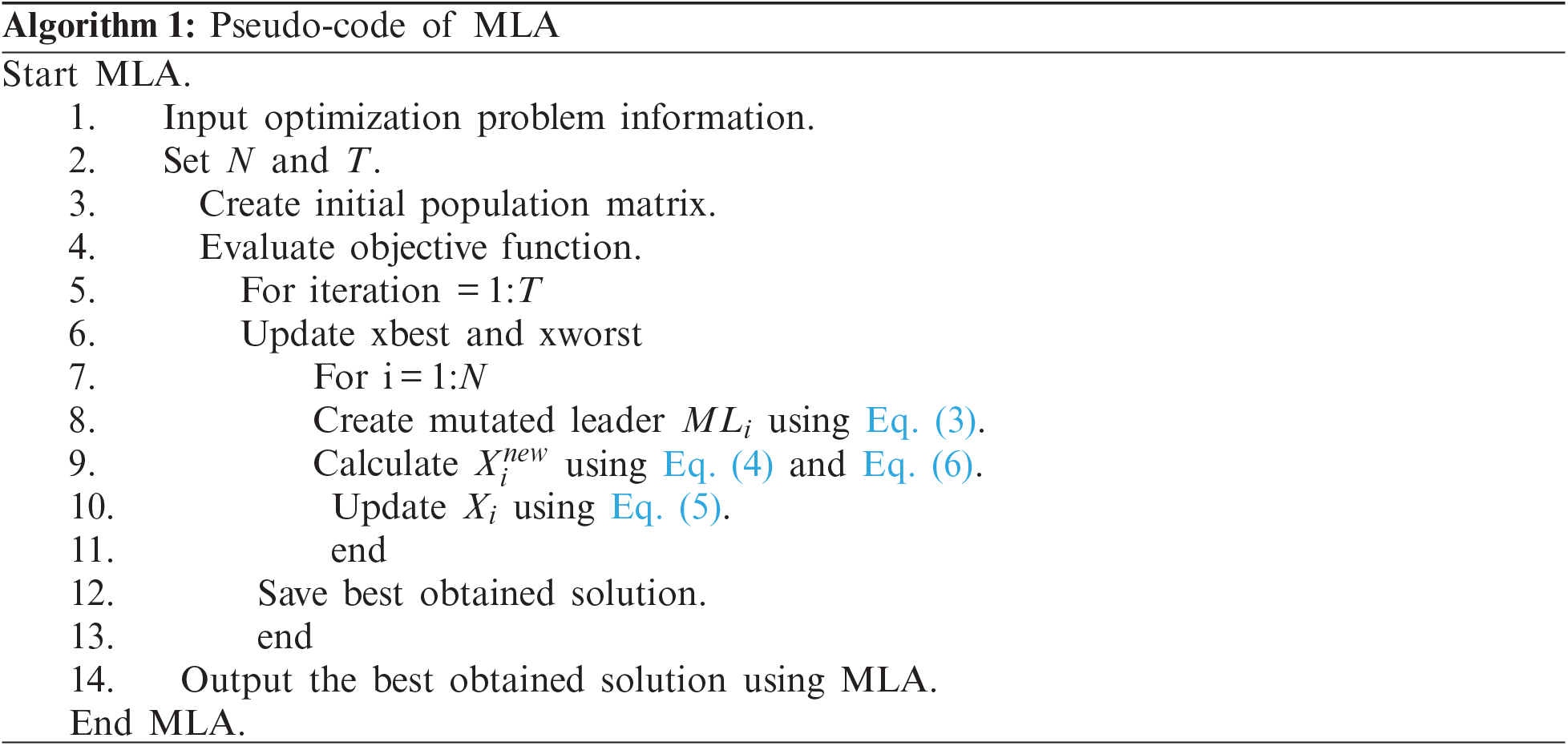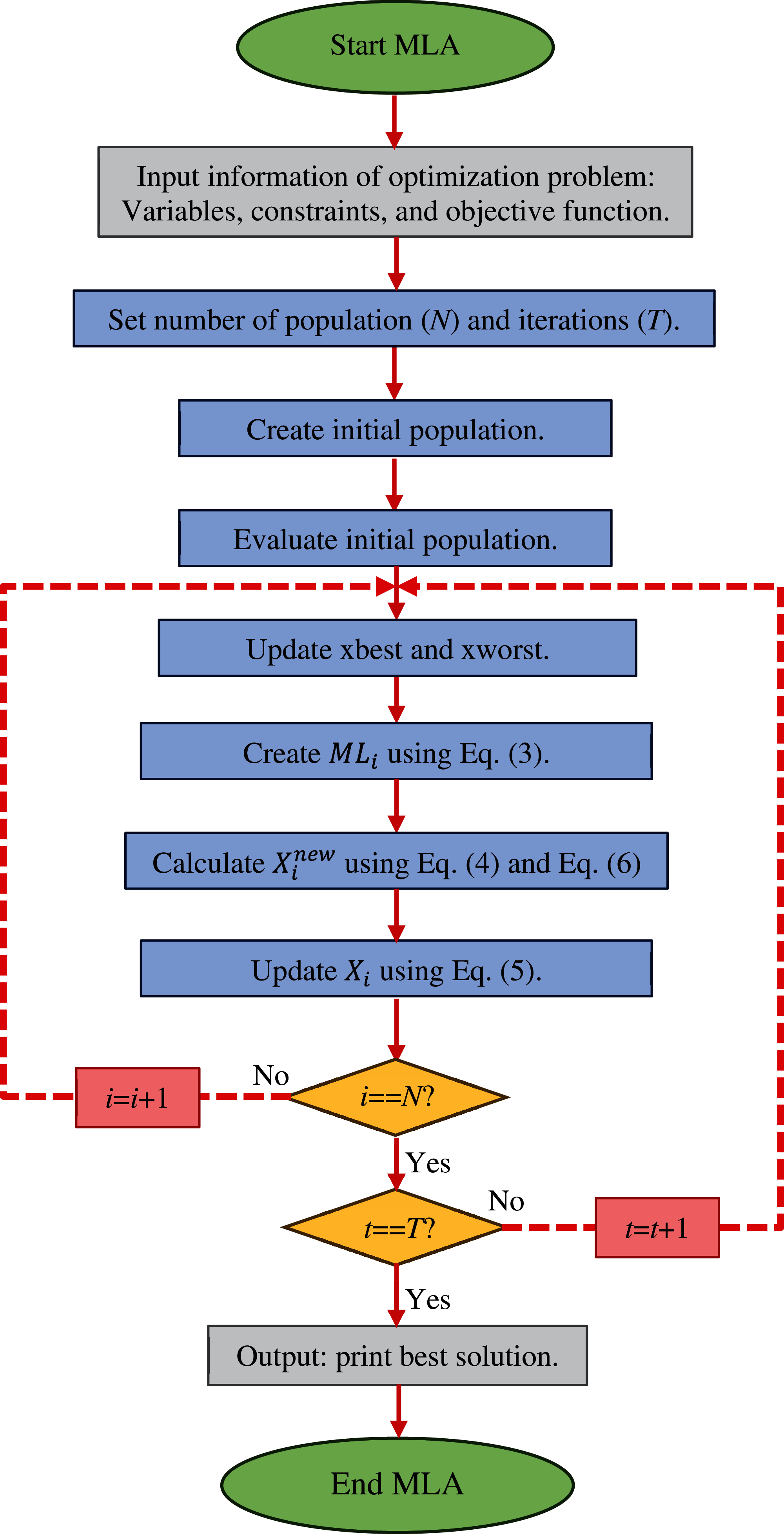Figure 1: Flowchart of MLA

4  Simulation Studies

In this section, simulation studies and performance analysis of the proposed MLA in solving optimization problems and quasi-optimal solutions are presented. The capability of the proposed MLA is tested on a standard set of twenty-three objective functions of different types of functions. Information about these standard functions is given in Appendix A in Tabs. A1A3. Also, the results of the proposed algorithm are compared with the performance of eight optimization algorithms including Particle Swarm Optimization (PSO) , Genetic Algorithm (GA) , Gravitation Search Algorithm (GSA) , Teaching-Learning-Based Optimization (TLBO) , Grey Wolf Optimizer (GWO) , Tunicate Swarm Algorithm (TSA) , Whale Optimization Algorithm (WOA) , and Marine Predators Algorithm (MPA) . At this stage, each of the optimization algorithms is simulated for 20 independent runs which the stop condition for iterations is reaching the number of iterations of the algorithm to 1000 per run. The results of the implementation of optimization algorithms on the objective functions are reported using the two criteria of the average of the obtained best solutions (ave) and the standard deviation of the obtained best solutions (std).

4.1 Evaluation of Unimodal Functions

The first group of functions considered to evaluate the performance of the proposed algorithm is of the type of unimodal functions, including seven functions F1 to F7. The results of optimization of F1 to F7 objective functions using the proposed MLA and eight compared algorithms are presented in Tab. 1. The proposed MLA is the best optimizer for the objective functions of F1, F2, F3, F4, F5, F6, and F7 by providing more appropriate solutions than the eight compared algorithms. In addition, MLA is able to provide the global optimal solution for F6. Comparison of the performance of optimization algorithms indicates that the proposed MLA has a high ability to solve unimodal optimization problems.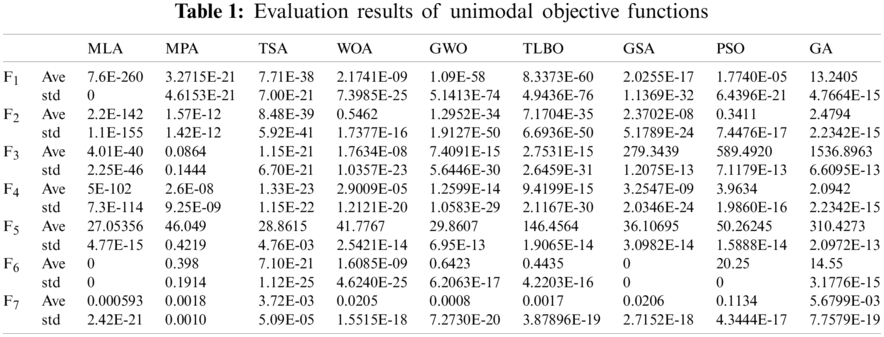4.2 Evaluation of High-Dimensional Functions

The second group of objective functions intended for analyzing the performance of the proposed MLA is selected from high-dimensional multimodal type, including six functions F8 to F13. The results of the implementation of the MLA and eight compared algorithms on these objective functions are presented in Tab. 2. MLA is the best optimizer for the objective functions F8, F10, and F12. In addition, the proposed MLA is able to provide the global optimal solution for F9 and F11. The analysis of the obtained results shows that the proposed MLA has an acceptable ability to optimize high-dimensional multimodal functions.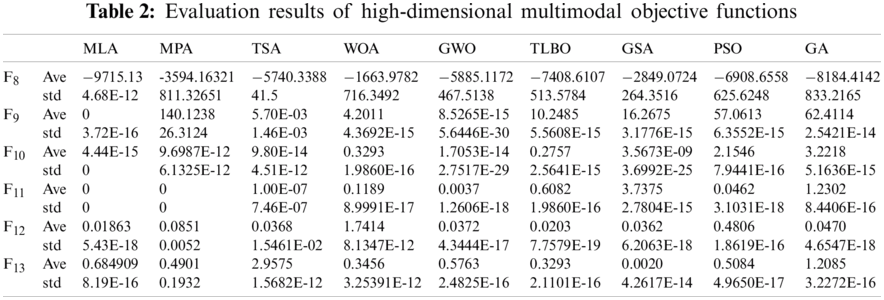4.3 Evaluation of Fixed-Dimensional Functions

The third group of objective functions is selected from fixed-dimensional multimodal type to evaluate the capability of optimization algorithms, including ten functions F14 to F23. The results of optimization of these objective functions using the proposed MLA and eight compared algorithms are presented in Tab. 3. The proposed MLA is the best optimizer for F15 and F16 functions and in other objective functions it has provided quasi-optimal solutions with less standard deviation. Comparison of the performance of the proposed MLA against the eight mentioned algorithms shows that the MLA has a high ability to solve multi-model problems and is more competitive than similar algorithms.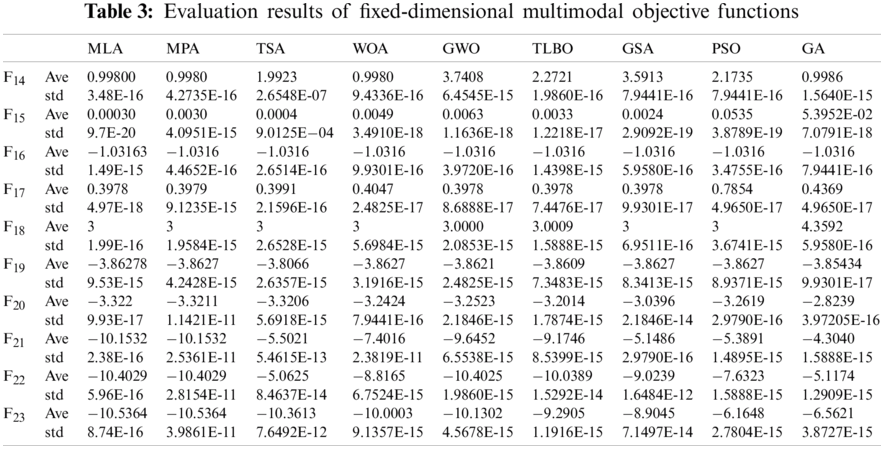4.4 Statistical Analysis

Presenting the results of implementation of optimization algorithms on optimization problems using two criteria of the average of the best solutions obtained and their standard deviation provides valuable information about the capabilities and quality of optimization algorithms. However, it is possible that even with a very low probability, the superiority of an algorithm occurred by chance. Therefore, in this subsection, in order to further analyze the performance of optimization algorithms, a statistical analysis on the results obtained from them is presented. In this regard, Wilcoxon rank sum test, which is a non-parametric statistical test, is used to specify whether the results obtained by the proposed MLA are different from compared eight algorithms in a statistically significant way.

A p-value indicates whether the performance difference of the given algorithm is statistically significant or not. The results of the statistical test obtained from the Wilcoxon rank sum test are reported in Tab. 4. Based on the analysis of the results presented in Tab. 4, it is clear that the proposed MLA in cases where the p-value is less than 0.05 is statistically different from the compared algorithm.4.5 Sensitivity Analysis

In this subsection, the sensitivity analysis of the proposed MLA to two parameters of maximum number of iterations and number of population members is discussed.

In the first stage, in order to analyze the sensitivity of the proposed MLA to the maximum number of iterations, the MLA in independent performances for the number of iterations of 100, 500, 800, and 1000 is implemented on twenty-three objective functions F1 to F23. The results of this simulation for different number of iterations and different functions are reported in Tab. 5. Based on the results obtained from this analysis, it is clear that with increasing the maximum number of iterations, the proposed MLA converges to more appropriate solutions and the values of the objective functions are reduced.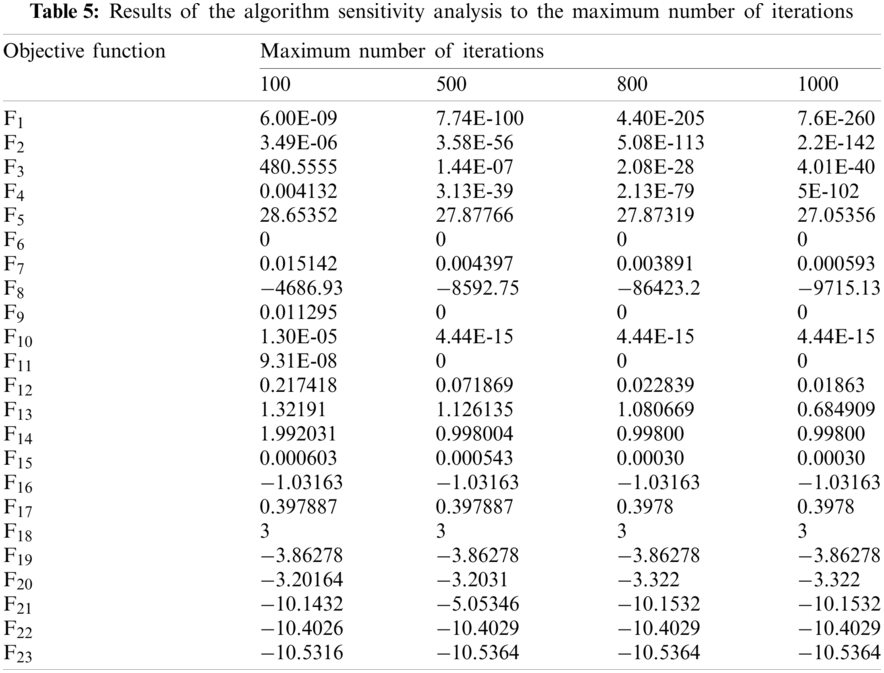In the second stage, in order to analyze the sensitivity of the proposed MLA to the number of population members, the proposed algorithm in independent performances for different populations with 20, 30, 50, and 80 members is implemented on twenty-three objective functions F1 to F23. The results of the sensitivity analysis of the MLA to the number of population members are presented in Tab. 6. What can be deduced from the simulation results of this analysis is that as the population members increase, the values of the objective functions decrease.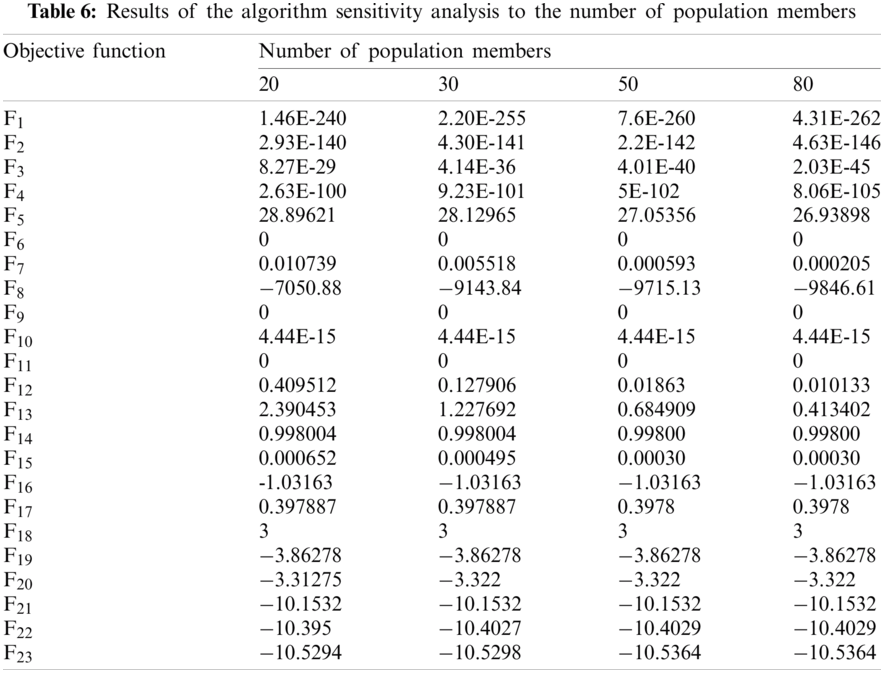5  Conclusions and Future Studies

Numerous optimization problems in science must be solved using appropriate methods. Optimization algorithms is one of the problem-solving methods from the group of stochastic methods that is able to provide appropriate solutions to optimization problems. In this paper, a new optimization algorithm called Mutated Leader Algorithm (MLA) was introduced and designed to solve optimization problems. Updating and guiding members of the population in the problem-solving space based on mutated leaders was the main idea in the proposed MLA design. The proposed MLA was mathematically modeled and its ability to solve optimization problems on twenty-three standard objective functions of various types was evaluated. Also, the results obtained from the MLA were compared with the results of eight algorithms named Genetic Algorithm (GA), Particle Swarm Optimization (PSO), Gravitational Search Algorithm (GSA), Teaching Learning-Based Optimization (TLBO), Grey Wolf Optimizer (GWO), Whale Optimization Algorithm (WOA), Marine Predators Algorithm (MPA), and Tunicate Swarm Algorithm (TSA). The optimization results showed the high ability of the proposed MLA to solve optimization problems. Also, analysis and comparison of the performance of the mentioned optimization algorithms against the MLA, showed that the proposed algorithm has a better performance and is much more competitive than similar algorithms.

For further study in the future, the authors offer several suggestions, including the design the binary and multi-objective versions of proposed MLA. In addition, the use of MLA in solving optimization problems in various fields as well as real life problems are other suggestions for future studies.

Funding Statement: PT (corresponding author) was supported by the Excellence project PřF UHK No. 2202/2020-2022 and Long-term development plan of UHK for year 2021, University of Hradec Králové, Czech Republic, https://www.uhk.cz/en/faculty-of-science/about-faculty/official-board/internal-regulations-and-governing-acts/governing-acts/deans-decision/2020#grant-competition-of-fos-uhk-excellence-for-2020.

Conflicts of Interest: The authors declare that they have no conflicts of interest to report regarding the present study.

References

1.  Y. Huang, T. Li, C. Luo, H. Fujita and S. J. Horng, “Dynamic variable precision rough set approach for probabilistic set-valued information systems,” Knowledge-Based Systems, vol. 122, pp. 131–147, 2017.

2.  L. C. Jiao, F. Shang, F. Wang and Y. Liu, “Fast semi-supervised clustering with enhanced spectral embedding,” Pattern Recognition, vol. 45, no. 12, pp. 4358–4369, 2012.

3.  I. Zelinka, V. Snasael and A. Abraham, “Handbook of optimization: from classical to modern approach,” in Computational Intelligence and Complexity, 1st ed., vol. 38. Heidelberg, Germany: Berlin Heidelberg: Springer-Verlag, pp. 1–1100, 2004.

4.  A. Beck, P. Stoica and J. Li, “Exact and approximate solutions of source localization problems,” IEEE Transactions on Signal Processing, vol. 56, no. 5, pp. 1770–1778, 2008.

5.  P. Singh and G. Dhiman, “A hybrid fuzzy time series forecasting model based on granular computing and bio-inspired optimization approaches,” Journal of Computational Science, vol. 27, pp. 370–385, 2018.

6.  G. Dhiman and V. Kumar, “Astrophysics inspired multi-objective approach for automatic clustering and feature selection in real-life environment,” Modern Physics Letters B, vol. 32, no. 31, pp. 1850385, 2018.

7.  R. Martí and G. Reinelt, “The linear ordering problem: Exact and heuristic methods in combinatorial optimization,” in Applied Mathematical Sciences, 1st ed., vol. 175. Heidelberg, Germany: Berlin, Heidelberg, Springer-Verlag, pp. 1–172, 2011.

8.  K. Kawamoto and K. Hirota, “Random scanning algorithm for tracking curves in binary image sequences,” International Journal of Intelligent Computing in Medical Sciences & Image Processing, vol. 2, no. 2, pp. 101–110, 2008.

9.  S. Russell and P. Norvig, “Artificial intelligence: A modern approach,” in Library of Congress Cataloging-in-Publication Data, 4st ed., vol. 1. Englewood Cliffs, New Jersey, USA: Alan Apt, pp. 1–932, 2002.

10. M. Dehghani, Z. Montazeri and O. P. Malik, “Energy commitment: A planning of energy carrier based on energy consumption,” Electrical Engineering & Electromechanics, no. 4, pp. 69–72, 2019.

11. M. Dehghani, M. Mardaneh, O. P. Malik, J. M. Guerrero, C. Sotelo et al., “Genetic algorithm for energy commitment in a power system supplied by multiple energy carriers,” Sustainability, vol. 12, no. 23, pp. 10053, 2020.

12. M. Dehghani, M. Mardaneh, O. P. Malik, J. M. Guerrero, R. Morales-Menendez et al., “Energy commitment for a power system supplied by multiple energy carriers system using following optimization algorithm,” Applied Sciences, vol. 10, no. 17, pp. 5862, 2020.

13. H. Rezk, A. Fathy, M. Aly and M. N. F. Ibrahim, “Energy management control strategy for renewable energy system based on spotted hyena optimizer,” Computers, Materials & Continua, vol. 67, no. 2, pp. 2271–2281, 2021.

14. A. Ehsanifar, M. Dehghani and M. Allahbakhshi, “Calculating the leakage inductance for transformer inter-turn fault detection using finite element method,” in Proc. of Iranian Conf. on Electrical Engineering (ICEETehran, Iran, pp. 1372–1377, 2017.

15. M. Dehghani, Z. Montazeri and O. Malik, “Optimal sizing and placement of capacitor banks and distributed generation in distribution systems using spring search algorithm,” International Journal of Emerging Electric Power Systems, vol. 21, no. 1, pp. 20190217, 2020.

16. M. Dehghani, Z. Montazeri, O. P. Malik, K. Al-Haddad, J. M. Guerrero et al., “A New methodology called dice game optimizer for capacitor placement in distribution systems,” Electrical Engineering & Electromechanics, vol. 1, pp. 61–64, 2020.

17. S. Dehbozorgi, A. Ehsanifar, Z. Montazeri, M. Dehghani and A. Seifi, “Line loss reduction and voltage profile improvement in radial distribution networks using battery energy storage system,” in Proc. of IEEE 4th Int. Conf. on Knowledge-Based Engineering and Innovation (KBEITehran, Iran, pp. 215–219, 2017.

18. Z. Montazeri and T. Niknam, “Optimal utilization of electrical energy from power plants based on final energy consumption using gravitational search algorithm,” Electrical Engineering & Electromechanics, vol. 4, pp. 70–73, 2018.

19. M. Dehghani, M. Mardaneh, Z. Montazeri, A. Ehsanifar, M. J. Ebadi et al., “Spring search algorithm for simultaneous placement of distributed generation and capacitors,” Electrical Engineering & Electromechanics, vol. 6, pp. 68–73, 2018.

20. M. Premkumar, R. Sowmya, P. Jangir, K. S. Nisar and M. Aldhaifallah, “A new metaheuristic optimization algorithms for brushless direct current wheel motor design problem,” Computers, Materials & Continua, vol. 67, no. 2, pp. 2227–2242, 2021.

21. M. Dehghani, Z. Montazeri, A. Ehsanifar, A. R. Seifi, M. J. Ebadi et al., “Planning of energy carriers based on final energy consumption using dynamic programming and particle swarm optimization,” Electrical Engineering & Electromechanics, vol. 5, pp. 62–71, 2018.

22. Z. Montazeri and T. Niknam, “Energy carriers management based on energy consumption,” in Proc. of IEEE 4th Int. Conf. on Knowledge-Based Engineering and Innovation (KBEITehran, Iran, pp. 539–543, 2017.

23. J. Kennedy and R. Eberhart, “Particle swarm optimization,” in Proc. of ICNN'95–Int. Conf. on Neural Networks, Perth, WA, Australia, pp. 1942–1948, 1995.

24. J. H. Holland, “Genetic algorithms,” Scientific American, vol. 267, no. 1, pp. 66–73, 1992.

25. E. Rashedi, H. Nezamabadi-Pour and S. Saryazdi, “GSA: A gravitational search algorithm,” Information Sciences, vol. 179, no. 13, pp. 2232–2248, 2009.

26. P. J. Van Laarhoven and E. H. Aarts, “Simulated annealing: Theory and applications,” in Mathematics and Its Applications, 1st ed., vol. 37. Germany: Springer Netherlands, pp. 7–15, 1987.

27. M. Dehghani, Z. Montazeri, A. Dehghani and A. Seifi, “Spring search algorithm: A new meta-heuristic optimization algorithm inspired by hooke's law,” in Proc. of IEEE 4th Int. Conf. on Knowledge-Based Engineering and Innovation (KBEITehran, Iran, pp. 210–214, 2017.

28. H. Du, X. Wu and J. Zhuang, “Small-world optimization algorithm for function optimization,” in Proc. of Int. Conf. on Natural Computation, Berlin, Heidelberg, pp. 264–273, 2006.

29. F. F. Moghaddam, R. F. Moghaddam and M. Cheriet, “Curved space optimization: A random search based on general relativity theory,” Arxiv Preprint Arxiv:1208.2214, 2012. https://arxiv.org/abs/1208.2214v1.

30. A. Kaveh and M. Khayatazad, “A new meta-heuristic method: Ray optimization,” Computers & Structures, vol. 112, pp. 283–294, 2012.

31. A. Hatamlou, “Black hole: A new heuristic optimization approach for data clustering,” Information Sciences, vol. 222, pp. 175–184, 2013.

32. M. Dehghani and H. Samet, “Momentum search algorithm: A new meta-heuristic optimization algorithm inspired by momentum conservation law,” SN Applied Sciences, vol. 2, no. 10, pp. 1–15, 2020.

33. B. Alatas, “ACROA: Artificial chemical reaction optimization algorithm for global optimization,” Expert Systems with Applications, vol. 38, no. 10, pp. 13170–13180, 2011.

34. A. Kaveh and S. Talatahari, “A novel heuristic optimization method: Charged system search,” Acta Mechanica, vol. 213, no. 3–4, pp. 267–289, 2010.

35. H. Shah-Hosseini, “Principal components analysis by the galaxy-based search algorithm: A novel metaheuristic for continuous optimisation,” International Journal of Computational Science and Engineering, vol. 6, no. 1–2, pp. 132–140, 2011.

36. M. H. Tayarani-N and M. R. Akbarzadeh-T, “Magnetic optimization algorithms a new synthesis,” in IEEE Congress on Evolutionary Computation (IEEE World Congress on Computational Intelligencepp. 2659–2664, Hong Kong, China, 2008.

37. D. Simon, “Biogeography-based optimization,” IEEE Transactions on Evolutionary Computation, vol. 12, no. 6, pp. 702–713, 2008.

38. R. Storn and K. Price, “Differential evolution–A simple and efficient heuristic for global optimization over continuous spaces,” Journal of Global Optimization, vol. 11, no. 4, pp. 341–359, 1997.

39. H. G. Beyer and H. P. Schwefel, “Evolution strategies–A comprehensive introduction,” Natural Computing, vol. 1, no. 1, pp. 3–52, 2002.

40. W. Banzhaf, P. Nordin, R. E. Keller and F. D. Francone, “Genetic programming: An introduction: On the automatic evolution of computer programs and its applications,” in Library of Congress Cataloging-in-Publication Data, 1st ed., vol. 27. San Francisco, CA, USA: Morgan Kaufmann Publishers, pp. 1–398, 1998.

41. M. Dorigo, M. Birattari and T. Stutzle, “Ant colony optimization,” IEEE Computational Intelligence Magazine, vol. 1, no. 4, pp. 28–39, 2006.

42. G. Dhiman and V. Kumar, “Emperor penguin optimizer: A bio-inspired algorithm for engineering problems,” Knowledge-Based Systems, vol. 159, pp. 20–50, 2018.

43. R. V. Rao, V. J. Savsani and D. Vakharia, “Teaching–learning-based optimization: A novel method for constrained mechanical design optimization problems,” Computer-Aided Design, vol. 43, no. 3, pp. 303–315, 2011.

44. S. Mirjalili, S. M. Mirjalili and A. Lewis, “Grey wolf optimizer,” Advances in Engineering Software, vol. 69, pp. 46–61, 2014.

45. M. Dehghani, M. Mardaneh and O. Malik, “FOA: ‘following’ optimization algorithm for solving power engineering optimization problems,” Journal of Operation and Automation in Power Engineering, vol. 8, no. 1, pp. 57–64, 2020.

46. M. Dehghani, Z. Montazeri and Š Hubálovský, “GMBO: Group mean-based optimizer for solving various optimization problems,” Mathematics, vol. 9, no. 11, pp. 1190, 2021.

47. M. Dehghani, M. Mardaneh, O. P. Malik and S. M. NouraeiPour, “DTO: Donkey theorem optimization,” In in Proc. of Iranian Conf. on Electrical Engineering (ICEEYazd, Iran, pp. 1855–1859, 2019.

48. S. Kaur, L. K. Awasthi, A. L. Sangal, and G. Dhiman, “Tunicate swarm algorithm: A new bio-inspired based metaheuristic paradigm for global optimization,” Engineering Applications of Artificial Intelligence, vol. 90, pp. 103541, 2020.

49. A. Faramarzi, M. Heidarinejad, S. Mirjalili and A. H. Gandomi, “Marine predators algorithm: A nature-inspired metaheuristic,” Expert Systems with Applications, vol. 152, pp. 113377, 2020.

50. S. Mirjalili and A. Lewis, “The whale optimization algorithm,” Advances in Engineering Software, vol. 95, pp. 51–67, 2016.

51. M. Dehghani, Z. Montazeri, S. Saremi, A. Dehghani, O. P. Malik et al., “HOGO: Hide objects game optimization,” International Journal of Intelligent Engineering and Systems, vol. 13, no. 4, pp. 216–225, 2020.

52. M. Dehghani, M. Mardaneh, J. M. Guerrero, O. Malik and V. Kumar, “Football game based optimization: An application to solve energy commitment problem,” International Journal of Intelligent Engineering and Systems, vol. 13, pp. 514–523, 2020.

53. M. Dehghani, Z. Montazeri, O. P. Malik, A. Ehsanifar and A. Dehghani, “OSA: Orientation search algorithm,” International Journal of Industrial Electronics, Control and Optimization, vol. 2, no. 2, pp. 99–112, 2019.

Appendix A. Objective functions

Information and details about the twenty-three standard objective functions used in the simulation section are given in Tabs. A1A3.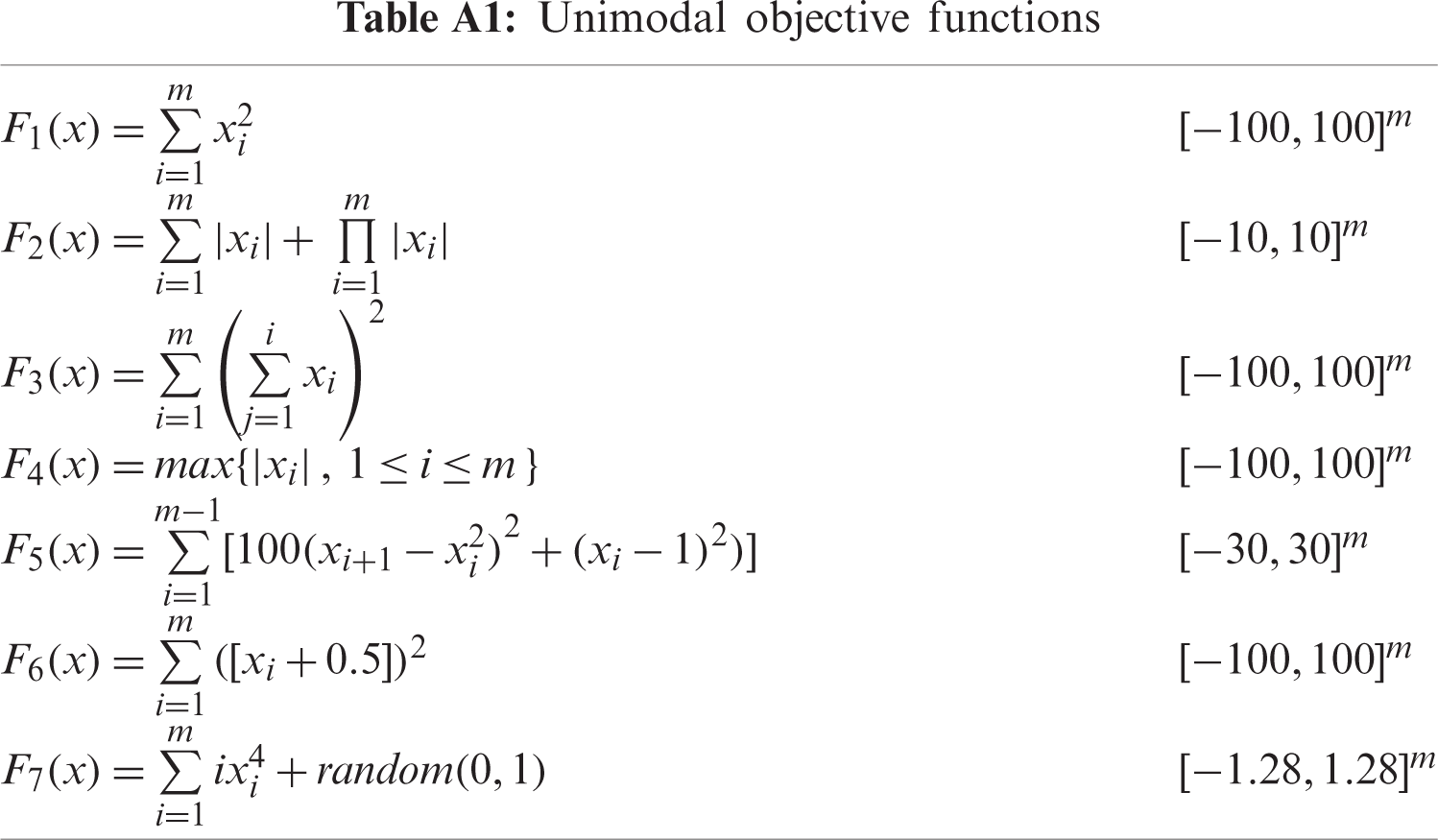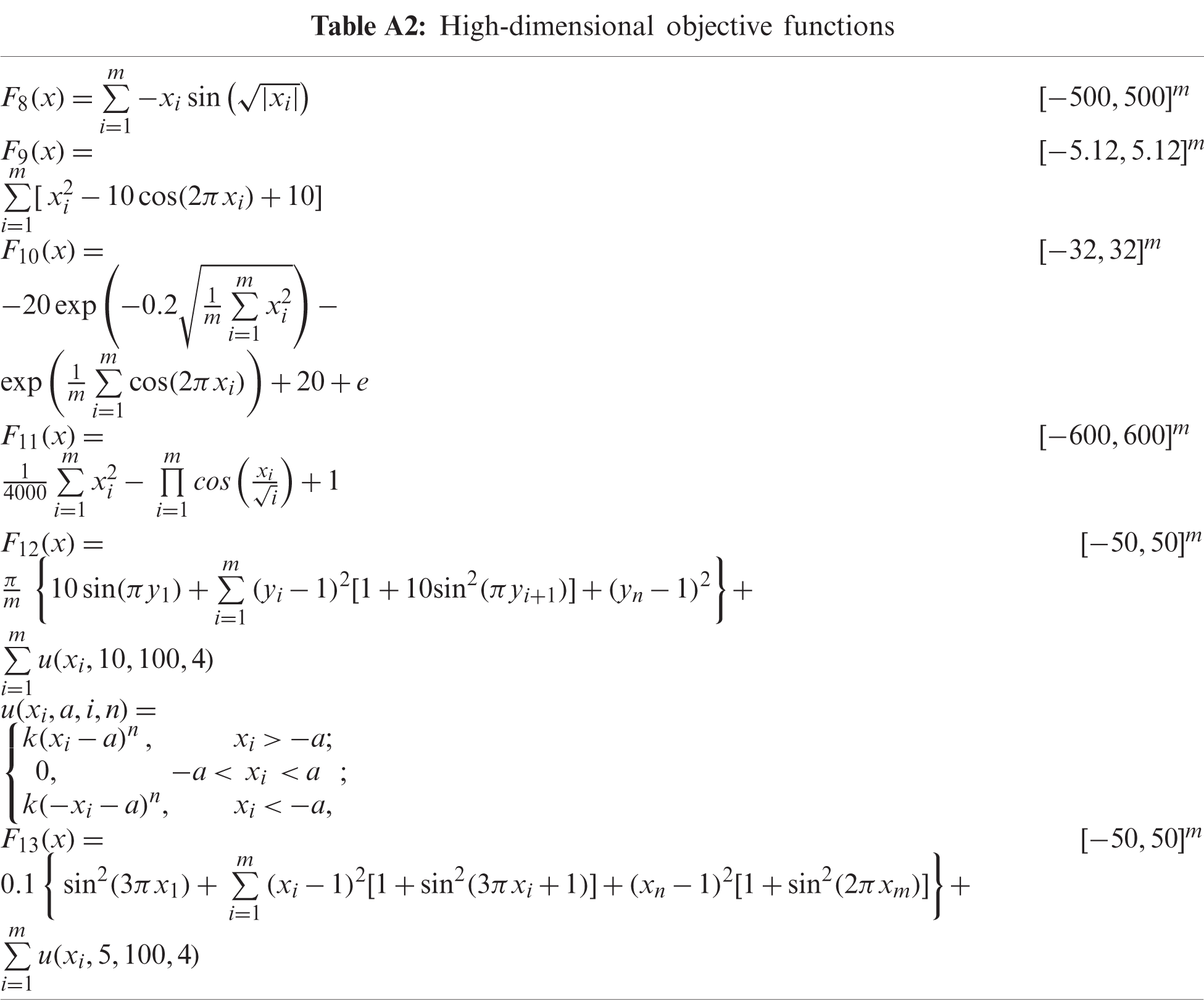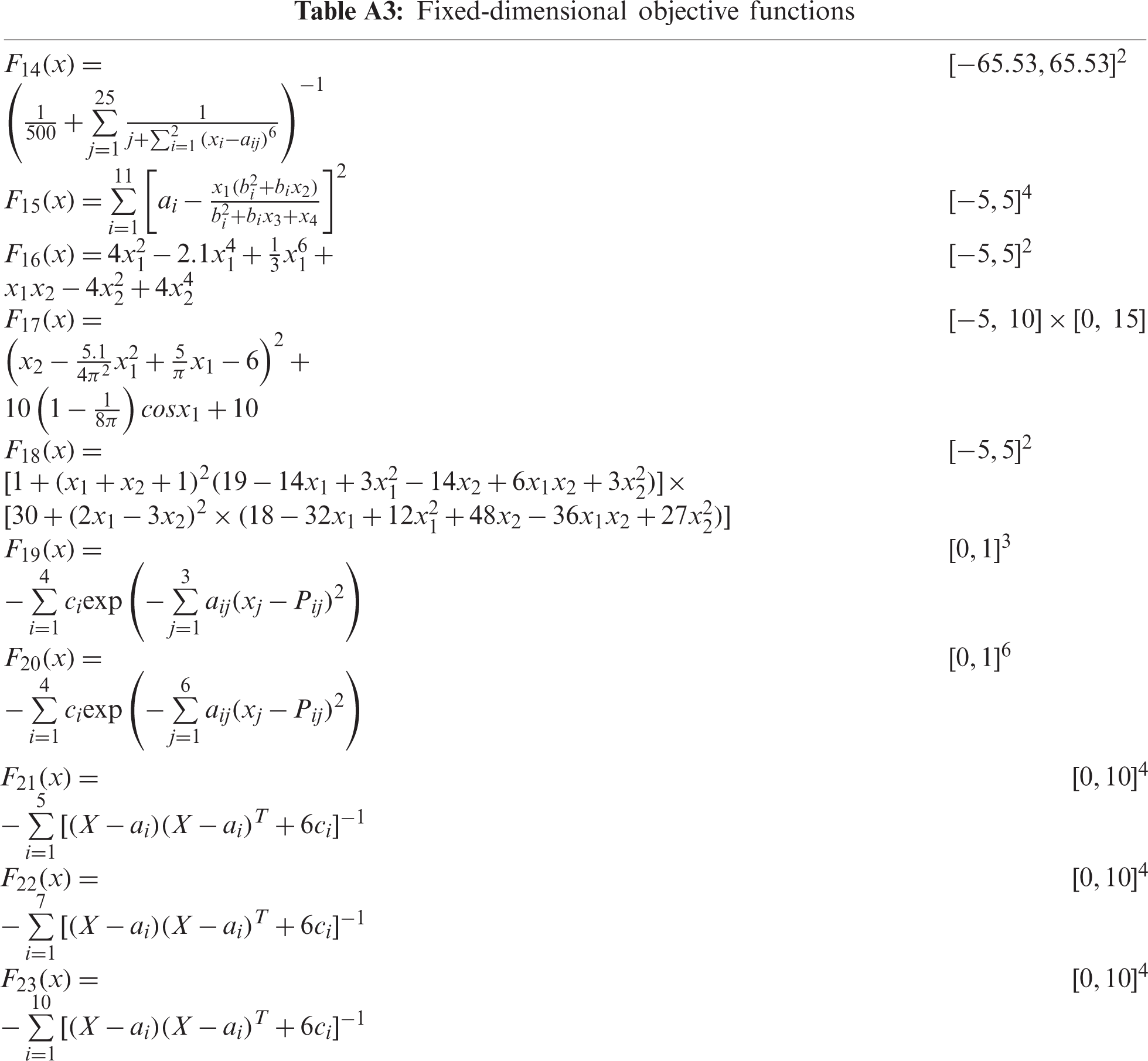This work is licensed under a Creative Commons Attribution 4.0 International License, which permits unrestricted use, distribution, and reproduction in any medium, provided the original work is properly cited.